# Exercise 2: Topic Model Inference in LDA¶

First, let's import the Python bindings for MeTA:

In :
import metapy


If you don't have metapy installed, you can install it with a

pip install metapy


on the command line on Linux, macOS, or Windows for either Python 2.7 or Python 3.x. (I will be using Python 3.6 in this tutorial.)

Double-check that you are running the latest version. Right now, that should be 0.2.10.

In :
metapy.__version__

Out:
'0.2.11'

Now, let's set MeTA to log to standard error so we can see progress output for long-running commands. (Only do this once, or you'll get double the output.)

In :
metapy.log_to_stderr()


Now, let's download all of the files we need for the tutorial.

In :
import urllib.request
import os
import tarfile

if not os.path.exists('sigir18-tutorial.tar.gz'):
urllib.request.urlretrieve('https://meta-toolkit.org/data/2018-06-25/sigir18-tutorial.tar.gz',
'sigir18-tutorial.tar.gz')

if not os.path.exists('data'):
with tarfile.open('sigir18-tutorial.tar.gz', 'r:gz') as files:
files.extractall()


The tutorial files come with a dataset consisting of four years of NIPS proceedings (full text): 2002, 2007, 2012, and 2017.

To start, we first want to understand what topics are being discussed in NIPS in these for years. To do that, we'll first index the dataset in the ForwardIndex format (we want to map documents to the terms that they contain).

In :
fidx = metapy.index.make_forward_index('nips.toml')

1530029662: [info]     Creating forward index: nips-idx/fwd (/tmp/pip-req-build-s8007td9/deps/meta/src/index/forward_index.cpp:239)
> Tokenizing Docs: [===================================>    ]  88% ETA 00:00:00
1530029664: [warning]  Empty document (id = 1435) generated! (/tmp/pip-req-build-s8007td9/deps/meta/src/index/forward_index.cpp:335)
> Tokenizing Docs: [========================================] 100% ETA 00:00:00
> Merging: [================================================] 100% ETA 00:00:00
1530029664: [info]     Done creating index: nips-idx/fwd (/tmp/pip-req-build-s8007td9/deps/meta/src/index/forward_index.cpp:278)


Now, let's load in all of the documents into memory so we can start to infer a topic model. I'm going to load them in as a MulticlassDataset because each document here has been associated with a label (the year it came from), but you could also load them in as just a standard Dataset with no associated labels if you don't plan to use them.

In :
dset = metapy.classify.MulticlassDataset(fidx)

 > Loading instances into memory: [==========================] 100% ETA 00:00:00


With the documents loaded into memory, we can start to run LDA inference on them to infer the topics and their coverage in each of the documents. There are several choices for inference algorithm in MeTA, so in general you can just pick your favorite. Here, I'm going to pick a parallelized version of Gibbs sampling.

The below will run the sampler for either 1000 iterations or until the log likelihood ($\log P(W \mid Z)$) stabilizes, whichever comes first. (If you want to disable the convergence checking and just run the sampler for a fixed number of iterations, you can add the parameter convergence=0.)

In :
model = metapy.topics.LDAParallelGibbs(docs=dset, num_topics=10, alpha=0.1, beta=0.1)
model.run(num_iters=1000)
model.save('lda-pgibbs-nips')

Initialization log likelihood (log P(W|Z)): -3.05507e+07
Iteration 1 log likelihood (log P(W|Z)): -3.05155e+07
Iteration 2 log likelihood (log P(W|Z)): -3.04318e+07
Iteration 3 log likelihood (log P(W|Z)): -3.03566e+07
Iteration 4 log likelihood (log P(W|Z)): -3.02892e+07
Iteration 5 log likelihood (log P(W|Z)): -3.0229e+07
Iteration 6 log likelihood (log P(W|Z)): -3.01748e+07
Iteration 7 log likelihood (log P(W|Z)): -3.0123e+07
Iteration 8 log likelihood (log P(W|Z)): -3.00742e+07
Iteration 9 log likelihood (log P(W|Z)): -3.00266e+07
Iteration 10 log likelihood (log P(W|Z)): -2.99788e+07
Iteration 11 log likelihood (log P(W|Z)): -2.99365e+07
Iteration 12 log likelihood (log P(W|Z)): -2.98953e+07
Iteration 13 log likelihood (log P(W|Z)): -2.98584e+07
Iteration 14 log likelihood (log P(W|Z)): -2.9823e+07
Iteration 15 log likelihood (log P(W|Z)): -2.97915e+07
Iteration 16 log likelihood (log P(W|Z)): -2.9764e+07
Iteration 17 log likelihood (log P(W|Z)): -2.97375e+07
Iteration 18 log likelihood (log P(W|Z)): -2.97107e+07
Iteration 19 log likelihood (log P(W|Z)): -2.96852e+07
Iteration 20 log likelihood (log P(W|Z)): -2.96599e+07
Iteration 21 log likelihood (log P(W|Z)): -2.96358e+07
Iteration 22 log likelihood (log P(W|Z)): -2.9616e+07
Iteration 23 log likelihood (log P(W|Z)): -2.95975e+07
Iteration 24 log likelihood (log P(W|Z)): -2.95787e+07
Iteration 25 log likelihood (log P(W|Z)): -2.95601e+07
Iteration 26 log likelihood (log P(W|Z)): -2.95423e+07
Iteration 27 log likelihood (log P(W|Z)): -2.95256e+07
Iteration 28 log likelihood (log P(W|Z)): -2.95089e+07
Iteration 29 log likelihood (log P(W|Z)): -2.94934e+07
Iteration 30 log likelihood (log P(W|Z)): -2.94771e+07
Iteration 31 log likelihood (log P(W|Z)): -2.94604e+07
Iteration 32 log likelihood (log P(W|Z)): -2.94411e+07
Iteration 33 log likelihood (log P(W|Z)): -2.94258e+07
Iteration 34 log likelihood (log P(W|Z)): -2.94121e+07
Iteration 35 log likelihood (log P(W|Z)): -2.93981e+07
Iteration 36 log likelihood (log P(W|Z)): -2.93842e+07
Iteration 37 log likelihood (log P(W|Z)): -2.93683e+07
Iteration 38 log likelihood (log P(W|Z)): -2.93535e+07
Iteration 39 log likelihood (log P(W|Z)): -2.93385e+07
Iteration 40 log likelihood (log P(W|Z)): -2.93255e+07
Iteration 41 log likelihood (log P(W|Z)): -2.93134e+07
Iteration 42 log likelihood (log P(W|Z)): -2.93024e+07
Iteration 43 log likelihood (log P(W|Z)): -2.92893e+07
Iteration 44 log likelihood (log P(W|Z)): -2.92773e+07
Iteration 45 log likelihood (log P(W|Z)): -2.92652e+07
Iteration 46 log likelihood (log P(W|Z)): -2.92528e+07
Iteration 47 log likelihood (log P(W|Z)): -2.92426e+07
Iteration 48 log likelihood (log P(W|Z)): -2.92319e+07
Iteration 49 log likelihood (log P(W|Z)): -2.92214e+07
Iteration 50 log likelihood (log P(W|Z)): -2.92125e+07
Iteration 51 log likelihood (log P(W|Z)): -2.92024e+07
Iteration 52 log likelihood (log P(W|Z)): -2.91915e+07
Iteration 53 log likelihood (log P(W|Z)): -2.91817e+07
Iteration 54 log likelihood (log P(W|Z)): -2.91718e+07
Iteration 55 log likelihood (log P(W|Z)): -2.91624e+07
Iteration 56 log likelihood (log P(W|Z)): -2.91524e+07
Iteration 57 log likelihood (log P(W|Z)): -2.91437e+07
Iteration 58 log likelihood (log P(W|Z)): -2.9136e+07
Iteration 59 log likelihood (log P(W|Z)): -2.91267e+07
Iteration 60 log likelihood (log P(W|Z)): -2.91166e+07
Iteration 61 log likelihood (log P(W|Z)): -2.91116e+07
Iteration 62 log likelihood (log P(W|Z)): -2.91032e+07
Iteration 63 log likelihood (log P(W|Z)): -2.90974e+07
Iteration 64 log likelihood (log P(W|Z)): -2.90928e+07
Iteration 65 log likelihood (log P(W|Z)): -2.90862e+07
Iteration 66 log likelihood (log P(W|Z)): -2.90802e+07
Iteration 67 log likelihood (log P(W|Z)): -2.9076e+07
Iteration 68 log likelihood (log P(W|Z)): -2.90701e+07
Iteration 69 log likelihood (log P(W|Z)): -2.90642e+07
Iteration 70 log likelihood (log P(W|Z)): -2.90588e+07
Iteration 71 log likelihood (log P(W|Z)): -2.90521e+07
Iteration 72 log likelihood (log P(W|Z)): -2.90461e+07
Iteration 73 log likelihood (log P(W|Z)): -2.90403e+07
Iteration 74 log likelihood (log P(W|Z)): -2.90336e+07
Iteration 75 log likelihood (log P(W|Z)): -2.90275e+07
Iteration 76 log likelihood (log P(W|Z)): -2.9024e+07
Iteration 77 log likelihood (log P(W|Z)): -2.90169e+07
Iteration 78 log likelihood (log P(W|Z)): -2.90139e+07
Iteration 79 log likelihood (log P(W|Z)): -2.90059e+07
Iteration 80 log likelihood (log P(W|Z)): -2.90029e+07
Iteration 81 log likelihood (log P(W|Z)): -2.89997e+07
Iteration 82 log likelihood (log P(W|Z)): -2.8994e+07
Iteration 83 log likelihood (log P(W|Z)): -2.89882e+07
Iteration 84 log likelihood (log P(W|Z)): -2.89821e+07
Iteration 85 log likelihood (log P(W|Z)): -2.89808e+07
Iteration 86 log likelihood (log P(W|Z)): -2.89763e+07
Iteration 87 log likelihood (log P(W|Z)): -2.89707e+07
Iteration 88 log likelihood (log P(W|Z)): -2.89659e+07
Iteration 89 log likelihood (log P(W|Z)): -2.89618e+07
Iteration 90 log likelihood (log P(W|Z)): -2.89592e+07
Iteration 91 log likelihood (log P(W|Z)): -2.89556e+07
Iteration 92 log likelihood (log P(W|Z)): -2.89521e+07
Iteration 93 log likelihood (log P(W|Z)): -2.89499e+07
Iteration 94 log likelihood (log P(W|Z)): -2.89452e+07
Iteration 95 log likelihood (log P(W|Z)): -2.8943e+07
Iteration 96 log likelihood (log P(W|Z)): -2.89395e+07
Iteration 97 log likelihood (log P(W|Z)): -2.89344e+07
Iteration 98 log likelihood (log P(W|Z)): -2.89316e+07
Iteration 99 log likelihood (log P(W|Z)): -2.89272e+07
Iteration 100 log likelihood (log P(W|Z)): -2.89221e+07
Iteration 101 log likelihood (log P(W|Z)): -2.89194e+07
Iteration 102 log likelihood (log P(W|Z)): -2.89157e+07
Iteration 103 log likelihood (log P(W|Z)): -2.89128e+07
Iteration 104 log likelihood (log P(W|Z)): -2.89098e+07
Iteration 105 log likelihood (log P(W|Z)): -2.89056e+07
Iteration 106 log likelihood (log P(W|Z)): -2.89036e+07
Iteration 107 log likelihood (log P(W|Z)): -2.88998e+07
Iteration 108 log likelihood (log P(W|Z)): -2.88973e+07
Iteration 109 log likelihood (log P(W|Z)): -2.88933e+07
Iteration 110 log likelihood (log P(W|Z)): -2.88906e+07
Iteration 111 log likelihood (log P(W|Z)): -2.88857e+07
Iteration 112 log likelihood (log P(W|Z)): -2.88837e+07
Iteration 113 log likelihood (log P(W|Z)): -2.88801e+07
Iteration 114 log likelihood (log P(W|Z)): -2.88774e+07
Iteration 115 log likelihood (log P(W|Z)): -2.8874e+07
Iteration 116 log likelihood (log P(W|Z)): -2.88712e+07
Iteration 117 log likelihood (log P(W|Z)): -2.88682e+07
Iteration 118 log likelihood (log P(W|Z)): -2.88675e+07
Iteration 119 log likelihood (log P(W|Z)): -2.88655e+07
Iteration 120 log likelihood (log P(W|Z)): -2.88631e+07
Iteration 121 log likelihood (log P(W|Z)): -2.88604e+07
Iteration 122 log likelihood (log P(W|Z)): -2.886e+07
Iteration 123 log likelihood (log P(W|Z)): -2.88581e+07
Iteration 124 log likelihood (log P(W|Z)): -2.88562e+07
Iteration 125 log likelihood (log P(W|Z)): -2.88539e+07
Iteration 126 log likelihood (log P(W|Z)): -2.88511e+07
Iteration 127 log likelihood (log P(W|Z)): -2.88496e+07
Iteration 128 log likelihood (log P(W|Z)): -2.88483e+07
Iteration 129 log likelihood (log P(W|Z)): -2.88485e+07
Iteration 130 log likelihood (log P(W|Z)): -2.88463e+07
Iteration 131 log likelihood (log P(W|Z)): -2.88444e+07
Iteration 132 log likelihood (log P(W|Z)): -2.8841e+07
Iteration 133 log likelihood (log P(W|Z)): -2.88389e+07
Iteration 134 log likelihood (log P(W|Z)): -2.8839e+07
Iteration 135 log likelihood (log P(W|Z)): -2.88364e+07
Iteration 136 log likelihood (log P(W|Z)): -2.88347e+07
Iteration 137 log likelihood (log P(W|Z)): -2.8835e+07
Iteration 138 log likelihood (log P(W|Z)): -2.88348e+07
Iteration 139 log likelihood (log P(W|Z)): -2.8833e+07
Iteration 140 log likelihood (log P(W|Z)): -2.88309e+07
Iteration 141 log likelihood (log P(W|Z)): -2.8828e+07
Iteration 142 log likelihood (log P(W|Z)): -2.8827e+07
Iteration 143 log likelihood (log P(W|Z)): -2.88244e+07
Iteration 144 log likelihood (log P(W|Z)): -2.88224e+07
Iteration 145 log likelihood (log P(W|Z)): -2.88207e+07
Iteration 146 log likelihood (log P(W|Z)): -2.88156e+07
Iteration 147 log likelihood (log P(W|Z)): -2.88159e+07
Iteration 148 log likelihood (log P(W|Z)): -2.88156e+07
Iteration 149 log likelihood (log P(W|Z)): -2.88144e+07
Iteration 150 log likelihood (log P(W|Z)): -2.88137e+07
Iteration 151 log likelihood (log P(W|Z)): -2.88135e+07
Iteration 152 log likelihood (log P(W|Z)): -2.8813e+07
Iteration 153 log likelihood (log P(W|Z)): -2.88128e+07
Iteration 154 log likelihood (log P(W|Z)): -2.88114e+07
Iteration 155 log likelihood (log P(W|Z)): -2.88099e+07
Iteration 156 log likelihood (log P(W|Z)): -2.88091e+07
Iteration 157 log likelihood (log P(W|Z)): -2.88062e+07
Iteration 158 log likelihood (log P(W|Z)): -2.88021e+07
Iteration 159 log likelihood (log P(W|Z)): -2.88032e+07
Iteration 160 log likelihood (log P(W|Z)): -2.88007e+07
Iteration 161 log likelihood (log P(W|Z)): -2.88005e+07
Iteration 162 log likelihood (log P(W|Z)): -2.87996e+07
Iteration 163 log likelihood (log P(W|Z)): -2.87982e+07
Iteration 164 log likelihood (log P(W|Z)): -2.87974e+07
Iteration 165 log likelihood (log P(W|Z)): -2.87959e+07
Iteration 166 log likelihood (log P(W|Z)): -2.8795e+07
Iteration 167 log likelihood (log P(W|Z)): -2.87936e+07
Iteration 168 log likelihood (log P(W|Z)): -2.87928e+07
Iteration 169 log likelihood (log P(W|Z)): -2.87938e+07
Iteration 170 log likelihood (log P(W|Z)): -2.87925e+07
Iteration 171 log likelihood (log P(W|Z)): -2.87933e+07
Iteration 172 log likelihood (log P(W|Z)): -2.87899e+07
Iteration 173 log likelihood (log P(W|Z)): -2.8791e+07
Iteration 174 log likelihood (log P(W|Z)): -2.8792e+07
Iteration 175 log likelihood (log P(W|Z)): -2.87905e+07
Iteration 176 log likelihood (log P(W|Z)): -2.8789e+07
Iteration 177 log likelihood (log P(W|Z)): -2.87893e+07
Iteration 178 log likelihood (log P(W|Z)): -2.87886e+07
Iteration 179 log likelihood (log P(W|Z)): -2.87889e+07
Iteration 180 log likelihood (log P(W|Z)): -2.87903e+07
Iteration 181 log likelihood (log P(W|Z)): -2.87883e+07
Iteration 182 log likelihood (log P(W|Z)): -2.87883e+07
Found convergence after 182 iterations!
1530029871: [info]     Finished maximum iterations, or found convergence! (/tmp/pip-req-build-s8007td9/deps/meta/src/topics/lda_gibbs.cpp:77)


Once the above converges, it will save the results to disk. We can load the results into memory for inspection by loading an instance of the TopicModel class:

In :
model = metapy.topics.TopicModel('lda-pgibbs-nips')

 > Loading topic term probabilities: [=======================] 100% ETA 00:00:00


What do the topics discussed in NIPS over the last two decades roughly look like?

In :
for topic in range(0, model.num_topics()):
print("Topic {}:".format(topic + 1))
for tid, val in model.top_k(topic, 10, metapy.topics.BLTermScorer(model)):
print("{}: {}".format(fidx.term_text(tid), val))
print("======\n")

Topic 1:
model: 0.047845971473469015
item: 0.036164145122550555
topic: 0.03582248886330763
document: 0.0301900041003383
latent: 0.029632168260620255
word: 0.02883241321447034
user: 0.0262748217085278
languag: 0.021573178212931057
lda: 0.014349119364181297
dirichlet: 0.013994334038283054
======

Topic 2:
neuron: 0.10418809366208093
spike: 0.07989410305538147
stimulus: 0.03402944466933788
respons: 0.027507819831649183
cell: 0.024650823093126214
signal: 0.023464992947839394
brain: 0.021329972080580163
time: 0.017871200820055985
fire: 0.017004659525601817
stimuli: 0.016437347395342597
======

Topic 3:
polici: 0.18494968655605182
action: 0.09615364214854322
reward: 0.08377584211666766
agent: 0.0808785827262104
game: 0.05217837257413512
state: 0.04843198689741257
reinforc: 0.04066073218825473
trajectori: 0.03331838788594098
mdp: 0.02327701848342051
player: 0.022277731661894288
======

Topic 4:
kernel: 0.058391015341538656
label: 0.05470488694787862
classifi: 0.04543920685948777
classif: 0.031377691511149046
featur: 0.025877748375414726
train: 0.02553568880708937
svm: 0.022467854412508627
loss: 0.02242496964410883
data: 0.01836611529415411
class: 0.015976898254645416
======

Topic 5:
posterior: 0.043363904458780314
estim: 0.039974266551518416
distribut: 0.037820339074187546
gaussian: 0.03719766917650302
model: 0.026377288657187144
densiti: 0.026100306065981422
log: 0.025795145745510784
bayesian: 0.025720736258683725
likelihood: 0.02534425739469013
infer: 0.023800936808848146
======

Topic 6:
imag: 0.1848253958028947
featur: 0.03939641238659535
pixel: 0.03318427554113301
object: 0.03283463933643374
video: 0.025197762764204847
detect: 0.024957985815931668
patch: 0.024620771066662606
visual: 0.02064621098389648
recognit: 0.02001959511406888
segment: 0.019845380852013594
======

Topic 7:
network: 0.07910535955554107
layer: 0.07722388990594499
train: 0.06835261643490363
deep: 0.055934507818227536
arxiv: 0.040912721761014216
gan: 0.036840912548190934
imag: 0.02695400262646877
neural: 0.02637093023830018
convolut: 0.025571579176695725
======

Topic 8:
regret: 0.06361698186025525
bound: 0.058111744797171745
algorithm: 0.03820927485218057
theorem: 0.033408446197696236
arm: 0.0277968207933802
bandit: 0.027590683375529772
xt: 0.024684500137442878
loss: 0.022575873415732153
lemma: 0.021536924307693606
submodular: 0.0213692020268514
======

Topic 9:
convex: 0.040845864136262344
matrix: 0.040525229678109884
norm: 0.025644169130572214
theorem: 0.019813100486113736
converg: 0.018802910828488756
algorithm: 0.01688950995760653
xk: 0.016387059495701076
spars: 0.016093131882315426
descent: 0.013754336933754541
======

Topic 10:
cluster: 0.08828989453659127
node: 0.08591088457917238
graph: 0.08147474857171028
tree: 0.04597631491614074
edg: 0.04420999696187278
algorithm: 0.01969405292165792
hash: 0.01941101971247532
partit: 0.016920807850431176
xi: 0.014332616516548316
network: 0.012698787363157476
======



# Exercise 3: Text Mining using Topic Models¶

## Topics over Time¶

An interesting "mining" question to ask on top of this is whether or not the topics used in NIPS have changed over time. Are certain topics exhibited only in the earlier years, or vice-versa?

To do this, let's take a look at the other output of LDA---the topic proportion vectors associated with each document. Since each document also has a label in our dataset, we can create plots for each topic to see the number of documents that mention a specific topic in a specific year, and to what degree.

We'll start by creating a simple dataset with pandas:

In :
import pandas as pd

data = []
for doc in dset:
proportions = model.topic_distribution(doc.id)
data.append([dset.label(doc)] + [proportions.probability(i) for i in range(0, model.num_topics())])
df = pd.DataFrame(data, columns=['label'] + ["Topic {}".format(i + 1) for i in range(0, model.num_topics())])


Now, let's plot the results. There a lot of ways to do this, but here I'm going to use a "swarm plot" so we can see where each and every document falls.

In :
%matplotlib inline
import seaborn as sns
import matplotlib.pyplot as plt

for i in range(0, model.num_topics()):
print("Topic {}".format(i + 1))
sns.swarmplot(data=df, x='label', y="Topic {}".format(i + 1))
plt.show()

Topic 1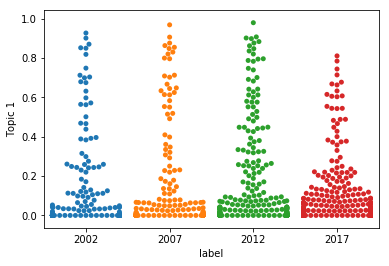Topic 2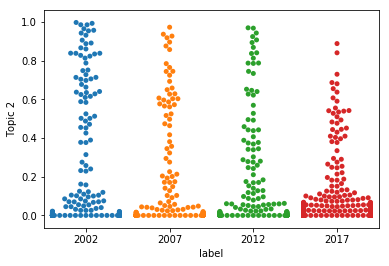Topic 3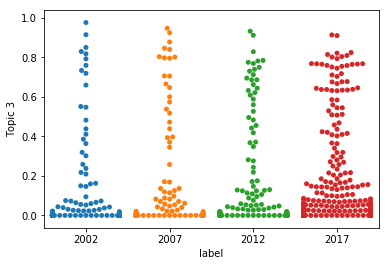Topic 4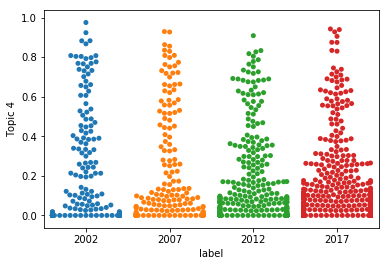Topic 5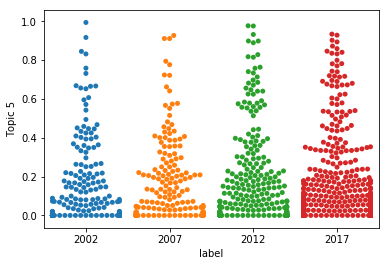Topic 6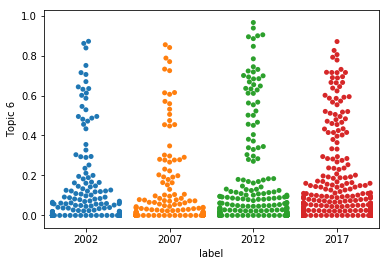Topic 7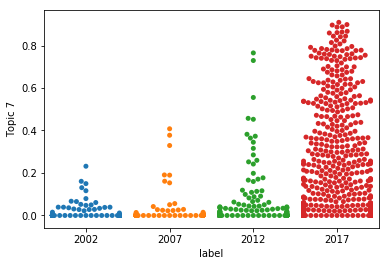Topic 8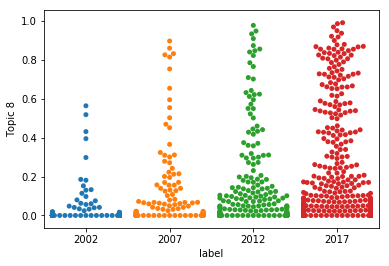Topic 9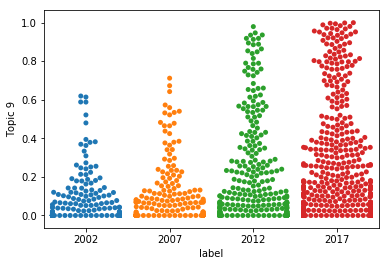Topic 10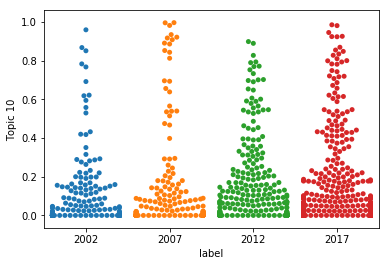## Topic Inference (Unseen Document)¶

Let's try to figure out what topics are mentioned in a previously unseen document.

In :
doc = metapy.index.Document()
with open('data/6589-scan-order-in-gibbs-sampling-models-in-which-it-matters-and-bounds-on-how-much.txt') as f:
print("{}...".format(doc.content()[0:500]))

Scan Order in Gibbs Sampling: Models in Which it
Matters and Bounds on How Much
Bryan He, Christopher De Sa, Ioannis Mitliagkas, and Christopher Ré
Stanford University
{bryanhe,cdesa,imit,chrismre}@stanford.edu

Abstract
Gibbs sampling is a Markov Chain Monte Carlo sampling technique that iteratively
samples variables from their conditional distributions. There are two common scan
orders for the variables: random scan and systematic scan. Due to the benefits
of locality in hardware, systematic s...


We first need to transform the unseen document into the same term-id space used by the topic model.

In :
dvec = fidx.tokenize(doc)


...and then we can create an inferencer on top of our topic model output to infer the topic coverage for this new document:

In :
inferencer = metapy.topics.GibbsInferencer('lda-pgibbs-nips.phi.bin', alpha=0.1)
props = inferencer.infer(dvec, max_iters=100, rng_seed=42)
print(props)

<metapy.stats.Multinomial {0: 0.125214, 1: 0.000036, 2: 0.240763, 3: 0.000036, 4: 0.161947, 5: 0.020007, 6: 0.000036, 7: 0.058167, 8: 0.020007, 9: 0.373787}>

 > Loading topic term probabilities: [=======================] 100% ETA 00:00:00


## Classification with Topic Features¶

The topic proportion vectors are also often used as input to a classifier. In our case, since we see some differences between the years 2002 and 2017 in terms of topical coverage, let's see if we can learn to separate documents that were written in 2002 from documents that were written in 2017 on the basis of their topic proportions alone.

In :
# First, create a lightweight view for shuffling
shuffled_view = metapy.classify.MulticlassDatasetView(dset)
shuffled_view.shuffle()

# this dataset will use unigram words as features
words_dset = metapy.classify.MulticlassDataset(
[doc for doc in shuffled_view if dset.label(doc) == "2002" or dset.label(doc) == "2017"],
dset.total_features(),
lambda doc: metapy.learn.FeatureVector(doc.weights),
lambda doc: dset.label(doc)
)

# this dataset will use topic proportions as features
topic_dset = metapy.classify.MulticlassDataset(
[doc for doc in shuffled_view if dset.label(doc) == "2002" or dset.label(doc) == "2017"],
model.num_topics(),
lambda doc: metapy.learn.FeatureVector((i, model.topic_probability(doc.id, i)) for i in range(0, model.num_topics())),
lambda doc: dset.label(doc)
)


We'll use a 50/50 training/test split setup.

In :
words_train = words_dset[0:int(len(words_dset)/2)]
words_test = words_dset[int(len(words_dset)/2):]

topics_train = topic_dset[0:int(len(topic_dset)/2)]
topics_test = topic_dset[int(len(topic_dset)/2):]

In :
def make_linear_svm(training):
return metapy.classify.OneVsAll(training, metapy.classify.SGD, loss_id='hinge')

words_sgd = make_linear_svm(words_train)
topics_sgd = make_linear_svm(topics_train)

print("Words:")
mtrx = words_sgd.test(words_test)
print(mtrx)
mtrx.print_stats()

print("======")
print("Topics:")
mtrx = topics_sgd.test(topics_test)
print(mtrx)
mtrx.print_stats()

Words:

2002     2017
------------------
2002 | 0.883    0.117
2017 | 0.0392   0.961

------------------------------------------------------------
Class       F1 Score    Precision   Recall      Class Dist
------------------------------------------------------------
2002        0.883       0.883       0.883       0.251
2017        0.961       0.961       0.961       0.749
------------------------------------------------------------
Total       0.941       0.941       0.941
------------------------------------------------------------
443 predictions attempted, overall accuracy: 0.941

======
Topics:

2002     2017
------------------
2002 | 0.613    0.387
2017 | 0.0753   0.925

------------------------------------------------------------
Class       F1 Score    Precision   Recall      Class Dist
------------------------------------------------------------
2002        0.667       0.731       0.613       0.251
2017        0.9         0.877       0.925       0.749
------------------------------------------------------------
Total       0.844       0.841       0.847
------------------------------------------------------------
443 predictions attempted, overall accuracy: 0.847



While we don't beat unigram words, we still do very well for a model that is only using 10 features compared to the tens of thousands used by the words model:

In :
fidx.unique_terms()

Out:
66479

We can also try a straight multiclass classification problem: given a document, predect the year from the topic proportions alone.

In :
topic_dset = metapy.classify.MulticlassDataset(
[doc for doc in shuffled_view],
model.num_topics(),
lambda doc: metapy.learn.FeatureVector((i, model.topic_probability(doc.id, i)) for i in range(0, model.num_topics())),
lambda doc: dset.label(doc)
)

words_train = shuffled_view[0:int(len(shuffled_view)/2)]
words_test = shuffled_view[int(len(shuffled_view)/2):]

topics_train = topic_dset[0:int(len(topic_dset)/2)]
topics_test = topic_dset[int(len(topic_dset)/2):]

In :
words_svm = make_linear_svm(words_train)
topics_svm = make_linear_svm(topics_train)

words_mtrx = words_svm.test(words_test)
topics_mtrx = topics_svm.test(topics_test)

print("Words:")
print(words_mtrx)
words_mtrx.print_stats()

print("========")
print("Topics:")
print(topics_mtrx)
topics_mtrx.print_stats()

Words:

2002     2007     2012     2017
------------------------------------
2002 | 0.667    0.176    0.0926   0.0648
2007 | 0.314    0.195    0.398    0.0932
2012 | 0.107    0.16     0.487    0.246
2017 | 0.0217   0.00619  0.0774   0.895

------------------------------------------------------------
Class       F1 Score    Precision   Recall      Class Dist
------------------------------------------------------------
2002        0.59        0.529       0.667       0.147
2007        0.24        0.311       0.195       0.16
2012        0.506       0.526       0.487       0.254
2017        0.855       0.819       0.895       0.439
------------------------------------------------------------
Total       0.633       0.62        0.645
------------------------------------------------------------
736 predictions attempted, overall accuracy: 0.645

========
Topics:

2002     2007     2012     2017
------------------------------------
2002 | 0.102    0.148    0.352    0.398
2007 | 0.161    0.153    0.297    0.39
2012 | 0.0695   0.107    0.203    0.62
2017 | 0.0031   0.031    0.0495   0.916

------------------------------------------------------------
Class       F1 Score    Precision   Recall      Class Dist
------------------------------------------------------------
2002        0.145       0.25        0.102       0.147
2007        0.198       0.281       0.153       0.16
2012        0.242       0.299       0.203       0.254
2017        0.718       0.591       0.916       0.439
------------------------------------------------------------
Total       0.452       0.417       0.493
------------------------------------------------------------
736 predictions attempted, overall accuracy: 0.493



This is quite a bit harder!